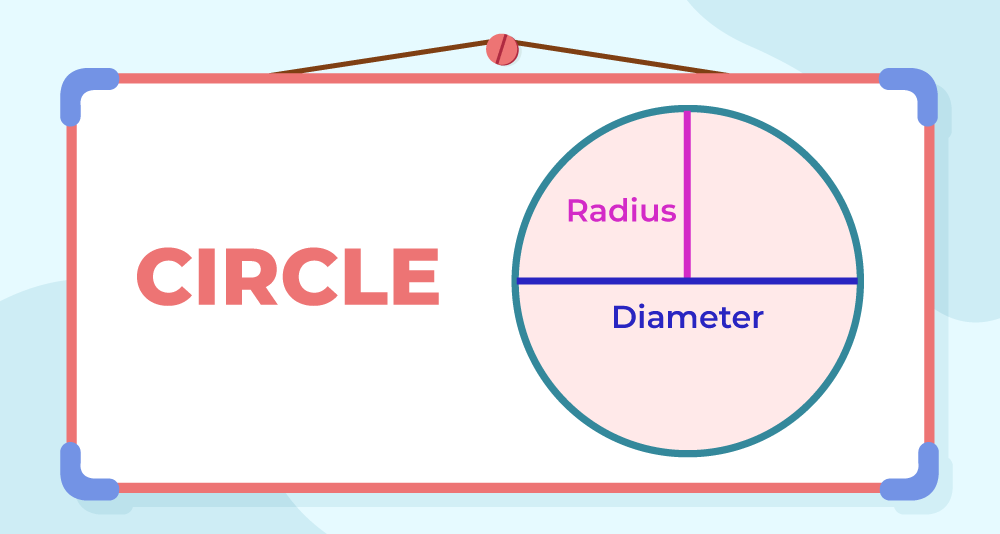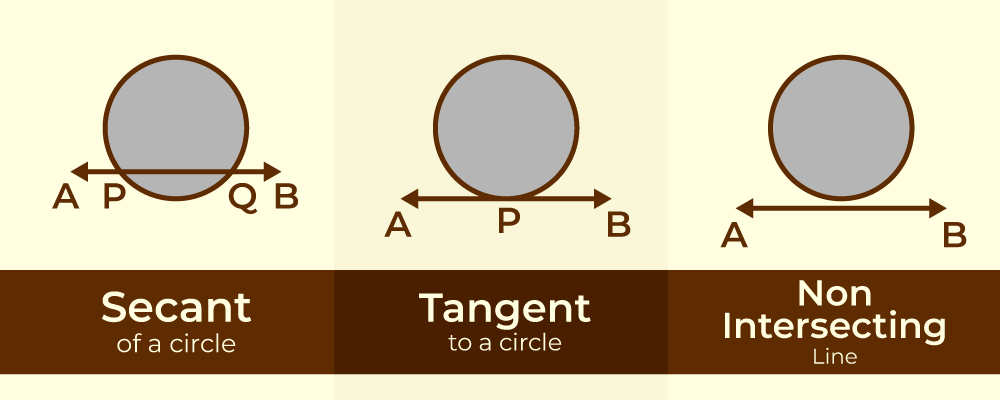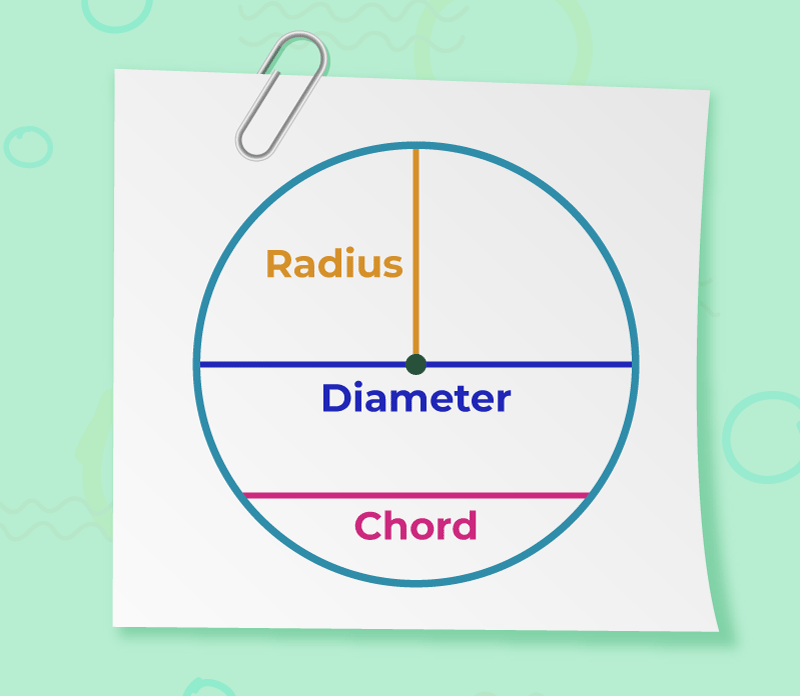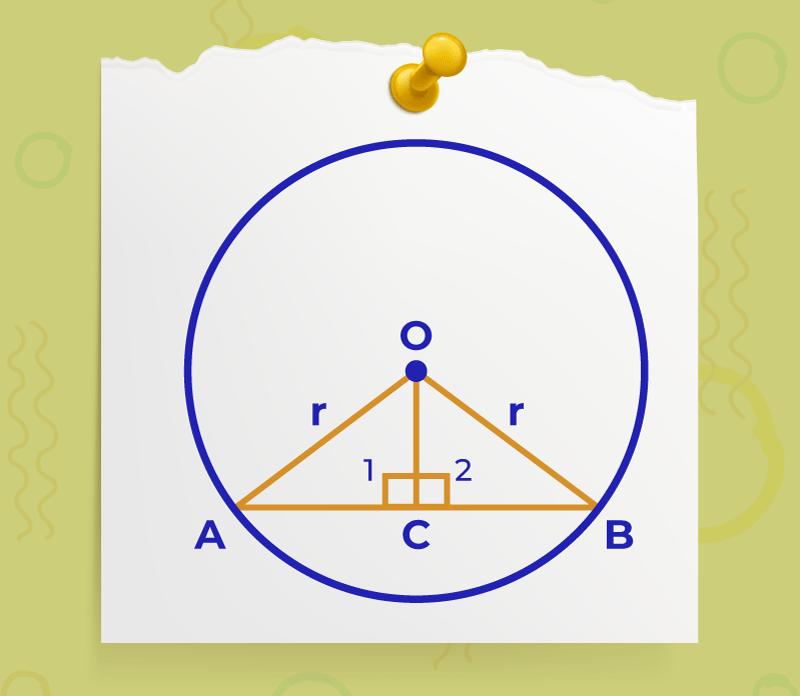Open In App
Related Articles
• CBSE Class 9 Maths Revision Notes
• CBSE Class 9 Maths Formulas
• NCERT Solutions for Class 9 Maths
• RD Sharma Class 9 Solutions

Radius of a circle is defined as the distance between the centre and any point in the circumference of the circle. It is always half of the diameter of a circle. Radius is used in the circle to find its,  area, and circumference, and is also used in other formulas. As we know that the locus of the point whose distance is always fixed from a fixed is the equation circle and the fixed distance is the radius of the circle.

A radius is a line segment that connects the centre of a circle or sphere to its periphery or boundaries. It is a component of circles and spheres that is commonly abbreviated as ‘r’. The plural of radius is “radii,” which is used when discussing more than one radius at the same time. The diameter of a circle or sphere is the longest line segment connecting all points on the opposite side of the centre, while the radius is half the length of the diameter.## Diameter of a Circle

The line joining two points in a circle and passing through the centre of the circle is called the Diameter of the circle. It is denoted by the symbol ‘d’ or ‘D’. The diameter of the circle is twice its radius.

• Diameter = 2 × Radius

Diameter is a chord of the circle which is the longest in nature.

Formulas of Circle (if Diameter is given)

Let the d represents the diameter of the circle then,

• Circumference of Circle = π(d)
• Area of Circle = π/4(d)2

Any line passing through the circle can be categorized into three categories,

• Intersecting Lines
• Tangent to a Circle
• Non-Intersecting Lines

Intersecting Lines

If a line touches the circle exactly two times then it is called Intersecting line. It is also called secant to the circle.

Tangent to a Circle

If a line touches the circle exactly one time then it is called a tangent to the circle.

Non-Intersecting Lines

If a line does not touch the circle then it is called Non-Intersecting Line.

The image given below shows the secant to a circle, tangent to a circle and non-intersecting lines to the circle.• Any line segment joining the centre of the circle to its circumference is called its radius.
• A line segment joining two points on the circumference of the circle is called the chord of the circle.
• The chord passing through the centre of the circle is called the diameter of the circle which is the longest chord of the circle.Radius of a circle is calculated with some specific formulas which are given below in the table

where,
d is the diameter,
C is the circumference,
A is the area.

The radius is equivalent to half of the diameter. i.e. Diameter = 2 × radius. The diameter is the largest chord of any circle.

The circumference of a circle is the measurement of a circle’s perimeter its formula is represented as

C = 2πr

where,
C is the circumference
π is constant with a value of 3.14

The radius of the circumference is also defined as the ratio of circumference to 2π. The radius formula using circumference is given by,

The space occupied by a circle is known as its area. The formula for the Area of a circle = πr2 square units defines the relation between the radius of any with its area. Here r represents the radius and the π constant is equal to 3.14159. The radius formula using circumference is given by,

Radius = √(Area / π) units

## How to Calculate the Radius of a Circle?

The radius of a circle can be found using the three basic radius formulas according to different conditions when the diameter is given when the area is given, and when the circumference is known. Let us use these formulas to find the radius of a circle.

• If the Diameter is known. Radius = Diameter / 2
• If Circumference is known. Radius = Circumference / 2π
• If Area is known. Radius = √(Area of the circle/π)

For example,

• When the diameter is given as 28 cm, then the radius is R = 28/2 = 14 cm
• When the circumference of a circle is given as 66 cm, then the radius is R = 66/2π = 10.5 cm
• When the area of a circle is given as 154 cm2, then the radius is R = √(154/π) = 7 cm

A sphere is a solid 3D shape. Radius of the Sphere is the distance between its centre and any point on its surface. Radius of a sphere is easily calculated when the volume of the sphere or the surface area of the sphere is given.

Radius of Sphere when Volume is Given

R = 3√{(3V) / 4π} units

where,
V represents the volume
π is constant with a value of approximately 3.14

Radius of Sphere when Surface Area is Given

R = √(A / 4π) units

where,
A represents the surface area
π is constant with a value of approximately 3.14

## Equation for Radius of Circle

The equation of a circle on the cartesian plane with centre (h, k) is given as (x − h)2 + (y − k)2 = r2. Where (x, y) is the locus of any point on the circumference of the circle and ‘r’ is the radius of the circle. If the origin (0,0) becomes the centre of the circle then its equation is given as x2 + y2 = r2. For finding the radius of the circle the following formula is used,

(Radius) r = √( x2 + y2 )

## Circle Theorems

### Theorem 1: Perpendicular line drawn from the centre of a circle to a chord bisects the chord.Given:

Chord AB and line segment OC is perpendicular to AB

To prove:

AC = BC

Construction:

Proof:

In ΔOAC and ΔOBC

∠OCA = ∠OCB (OC is perpendicular to AB)

OA = OB     (Radii of the same circle)

OC = OC     (Common Side)

So, by RHS congruence criterion ΔOAC ≅ ΔOBC

Thus, AC = CB (By CPCT)

Converse of the above theorem is also true.

### Theorem 2: Line drawn through the centre of the circle to bisect a chord is perpendicular to the chord.

(For Image reference see the Image used above.)

Given:

C is the midpoint of the chord AB of the circle with the centre of the circle at O

To prove:

OC is perpendicular to AB

Construction:

Join radii OA and OB also join OC

Proof:

In ∆OAC and ∆OBC

AC = BC (Given)

OA = OB (Radii of the same circle)

OC = OC (Common)

By SSS congruency criterion ∆OAC ≅ ∆OBC

∠1 = ∠2 (By CPCT)…(1)

∠1 + ∠2 = 180° (Linear pair angles)…(2)

Solving eq(1) and (2)

∠1 = ∠2 = 90°

Thus, OC is perpendicular to AB

Also, Check

## Solved Example on Radius Formulas

Example 1: Find the radius of the circle whose diameter is 18 cm.

Solution:

Given, Diameter of the circle = d = 18 cm

Radius of the circle by using diameter,

= 18 ⁄ 2 cm
= 9 cm

Hence, the radius of circle is 9 cm.

Example 2: Find the radius of a circle with a circumference of 14 cm.

Solution:

The radius of the circle with a circumference of 14 cm can be calculated by using the formula,

r = C / 2π

r = 14 / 2π {value of π = 22/7}

r = (14 × 7) / (2 × 22)

r = 98 / 44

r = 2.22 cm

Therefore, the radius of the given circle is 2.22 cm

Example 3: Find the area and the circumference of a circle whose radius is 12 cm. (Take the value of π = 3.14)

Solution:

Area of circle = π r2
= 3.14  × (12)2

A = 452.6 cm2

Now Circumference of circle,

C = 2πr
C = 2 × 3.14 × 12

Circumference = 75.36 cm

Therefore the area of circle is  452.6 cm2 and circumference of circle is 75.36 cm

Example 4: What is the radius of the circle if the area is 42 m2?

Solution:

Given: Area of circle, A = 42 m2.

The radius of the circle using area can be calculated by the formula, radius = √(A/π).

r = √(42/π) m

r = √{(42 × 7)/(22)} m

r = √294/22 m

r = 13.36 m

So, the radius of the circle is 13.36 m

Example 5: Find the diameter of a circle, given that area of a circle, is numerically equal to twice its circumference.

Given,

Area of the circle = 2 × circumference

We Know,

Area of the circle = π r2
Circumference = 2πr

Therefore,

π r2 = 2×2×π×r

r = 4

Therefore,

diameter = 2 × 4
= 8 units

## FAQs on Radius of Circle

### Q1: What radius of a circle?

The line joining the centre of the circle to any point in its circumference is called the radius of the circle. It is denoted by ‘r’ or ‘R’

### Q2: How many radii can be drawn in a circle?

A circle can have infinite radii drawn inside it.